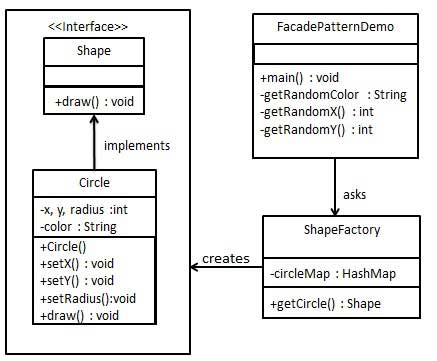# 享元模式

2021-11-26 16:48 更新

## 实现

ShapeFactory 有一个 CircleHashMap，其中键名为 Circle 对象的颜色。无论何时接收到请求，都会创建一个特定颜色的圆。ShapeFactory 检查它的 HashMap 中的 circle 对象，如果找到 Circle 对象，则返回该对象，否则将创建一个存储在 hashmap 中以备后续使用的新对象，并把该对象返回到客户端。

FlyWeightPatternDemo，我们的演示类使用 ShapeFactory 来获取 Shape 对象。它将向 ShapeFactory 传递信息（red / green / blue/ black / white），以便获取它所需对象的颜色。### 步骤 1

Shape.java

``````public interface Shape {
void draw();
}
``````

### 步骤 2

Circle.java

``````public class Circle implements Shape {
private String color;
private int x;
private int y;

public Circle(String color){
this.color = color;
}

public void setX(int x) {
this.x = x;
}

public void setY(int y) {
this.y = y;
}

}

@Override
public void draw() {
System.out.println("Circle: Draw() [Color : " + color
+", x : " + x +", y :" + y +", radius :" + radius);
}
}
``````

### 步骤 3

ShapeFactory.java

``````import java.util.HashMap;

public class ShapeFactory {
private static final HashMap<String, Shape> circleMap = new HashMap();

public static Shape getCircle(String color) {
Circle circle = (Circle)circleMap.get(color);

if(circle == null) {
circle = new Circle(color);
circleMap.put(color, circle);
System.out.println("Creating circle of color : " + color);
}
return circle;
}
}
``````

### 步骤 4

FlyweightPatternDemo.java

``````public class FlyweightPatternDemo {
private static final String colors[] =
{ "Red", "Green", "Blue", "White", "Black" };
public static void main(String[] args) {
for(int i=0; i < 20; ++i) {
Circle circle = (Circle)ShapeFactory.getCircle(getRandomColor());
circle.setX(getRandomX());
circle.setY(getRandomY());
circle.draw();
}
}
private static String getRandomColor() {
return colors[(int)(Math.random()*colors.length)];
}
private static int getRandomX() {
return (int)(Math.random()*100 );
}
private static int getRandomY() {
return (int)(Math.random()*100);
}
} ``````

### 步骤 5

``````Creating circle of color : Black
Circle: Draw() [Color : Black, x : 36, y :71, radius :100
Creating circle of color : Green
Circle: Draw() [Color : Green, x : 27, y :27, radius :100
Creating circle of color : White
Circle: Draw() [Color : White, x : 64, y :10, radius :100
Creating circle of color : Red
Circle: Draw() [Color : Red, x : 15, y :44, radius :100
Circle: Draw() [Color : Green, x : 19, y :10, radius :100
Circle: Draw() [Color : Green, x : 94, y :32, radius :100
Circle: Draw() [Color : White, x : 69, y :98, radius :100
Creating circle of color : Blue
Circle: Draw() [Color : Blue, x : 13, y :4, radius :100
Circle: Draw() [Color : Green, x : 21, y :21, radius :100
Circle: Draw() [Color : Blue, x : 55, y :86, radius :100
Circle: Draw() [Color : White, x : 90, y :70, radius :100
Circle: Draw() [Color : Green, x : 78, y :3, radius :100
Circle: Draw() [Color : Green, x : 64, y :89, radius :100
Circle: Draw() [Color : Blue, x : 3, y :91, radius :100
Circle: Draw() [Color : Blue, x : 62, y :82, radius :100
Circle: Draw() [Color : Green, x : 97, y :61, radius :100
Circle: Draw() [Color : Green, x : 86, y :12, radius :100
Circle: Draw() [Color : Green, x : 38, y :93, radius :100
Circle: Draw() [Color : Red, x : 76, y :82, radius :100
Circle: Draw() [Color : Blue, x : 95, y :82, radius :100
``````

App下载